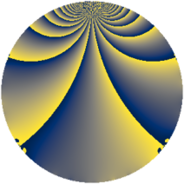# Properties

 Label 3549.2.bhLevel $3549$ Weight $2$ Character orbit 3549.bh Rep. character $\chi_{3549}(677,\cdot)$ Character field $\Q(\zeta_{6})$ Dimension $782$ Sturm bound $970$

# Related objects

## Defining parameters

 Level: $$N$$ $$=$$ $$3549 = 3 \cdot 7 \cdot 13^{2}$$ Weight: $$k$$ $$=$$ $$2$$ Character orbit: $$[\chi]$$ $$=$$ 3549.bh (of order $$6$$ and degree $$2$$) Character conductor: $$\operatorname{cond}(\chi)$$ $$=$$ $$21$$ Character field: $$\Q(\zeta_{6})$$ Sturm bound: $$970$$

## Dimensions

The following table gives the dimensions of various subspaces of $$M_{2}(3549, [\chi])$$.

Total New Old
Modular forms 1028 870 158
Cusp forms 916 782 134
Eisenstein series 112 88 24

## Trace form

 $$782q + 3q^{3} + 370q^{4} + 3q^{7} + q^{9} + O(q^{10})$$ $$782q + 3q^{3} + 370q^{4} + 3q^{7} + q^{9} + 12q^{10} + 24q^{12} - 12q^{15} - 332q^{16} + 10q^{18} + 9q^{19} - 4q^{21} + 8q^{22} - 36q^{24} - 297q^{25} + 12q^{28} + 40q^{30} - 27q^{31} - 4q^{36} + q^{37} - 36q^{40} - 70q^{42} + 42q^{43} + 6q^{45} + 48q^{46} - 31q^{49} + 22q^{51} + 54q^{54} - 10q^{57} + 12q^{58} - 16q^{60} + 60q^{61} + 71q^{63} - 480q^{64} + 12q^{66} - 21q^{67} + 4q^{70} - 62q^{72} - 21q^{73} - 57q^{75} + 23q^{79} - 27q^{81} - 216q^{82} + 24q^{84} - 64q^{85} + 48q^{87} - 44q^{88} + 53q^{93} - 72q^{94} - 66q^{96} + 68q^{99} + O(q^{100})$$

## Decomposition of $$S_{2}^{\mathrm{new}}(3549, [\chi])$$ into newform subspaces

The newforms in this space have not yet been added to the LMFDB.

## Decomposition of $$S_{2}^{\mathrm{old}}(3549, [\chi])$$ into lower level spaces

$$S_{2}^{\mathrm{old}}(3549, [\chi]) \cong$$ $$S_{2}^{\mathrm{new}}(21, [\chi])$$$$^{\oplus 3}$$$$\oplus$$$$S_{2}^{\mathrm{new}}(273, [\chi])$$$$^{\oplus 2}$$